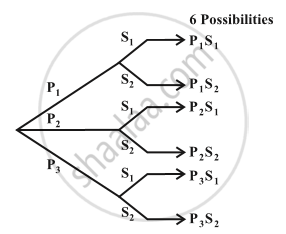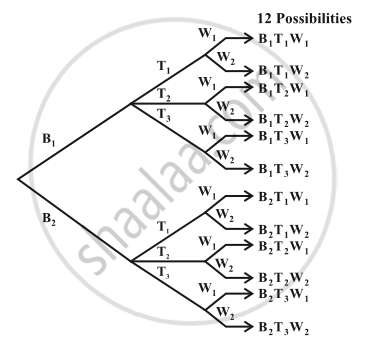# Fundamental Principle of Counting

#### notes

Let us consider the following problem. Mohan has 3 pants and 2 shirts. How many different pairs of a pant and a shirt, can he dress up with? There are 3 ways in which a pant can be chosen, because there are 3 pants available. Similarly, a shirt can be chosen in 2 ways. For every choice of a pant, there are 2 choices of a shirt. Therefore, there are 3 × 2 = 6 pairs of a pant and a shirt.
Let us name the three pants as P_1, P_2 , P_3  and the two shirts as S_1, S_2. Then, these six possibilities can be illustrated in the Fig.Let us consider another problem of the same type.
Sabnam has 2 school bags, 3 tiffin boxes and 2 water bottles. In how many ways can she carry these items (choosing one each).
A school  bag can be chosen in 2 different ways. After a school bag is chosen, a tiffin box can be chosen in 3 different ways. Hence, there are 2 × 3 = 6 pairs of school bag and a tiffin box. For each of these pairs a water bottle can be chosen in 2 different ways. Hence, there are 6 × 2 = 12 different ways in which, Sabnam can carry these items to school. If we name the 2 school bags as B_1, B_2, the three tiffin boxes as T_1, T_2, T_3 and the two water bottles as W_1, W_2, these possibilities can be illustrated in the Fig.“If an event can occur in m different ways, following which another event can occur in n different ways, then the total number of occurrence of the events in the given order is m×n.”
In the first problem, the required number of ways of wearing a pant and a shirt was the number of different ways of the occurence of the following events in succession:
(i) the event of choosing a pant
(ii) the event of choosing a shirt.
In the second problem, the required number of ways was the number of different ways of the occurence of the following events in succession:
(i) the event of choosing a school bag
(ii) the event of choosing a tiffin box
(iii) the event of choosing a water bottle.
Here, in both the cases, the events in each problem could occur in various possible orders. But, we have to choose any one of the possible orders and count the number of different ways of the occurence of the events in this chosen order.

If you would like to contribute notes or other learning material, please submit them using the button below.

### Shaalaa.com

Fundamental Principle of Counting [00:07:00]
S
0%